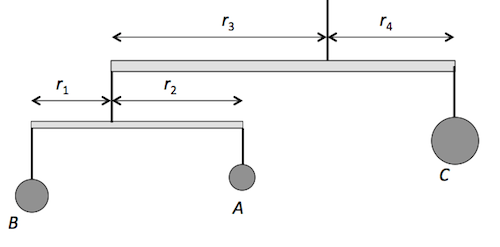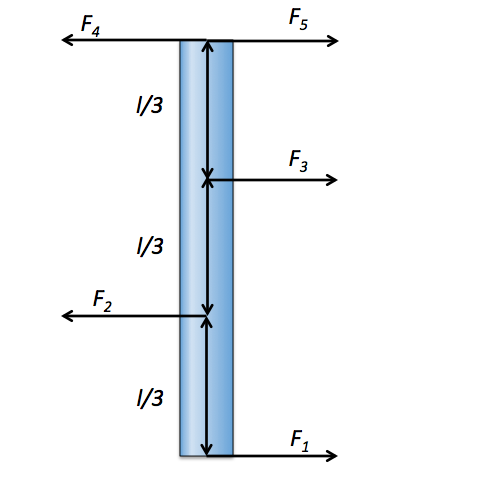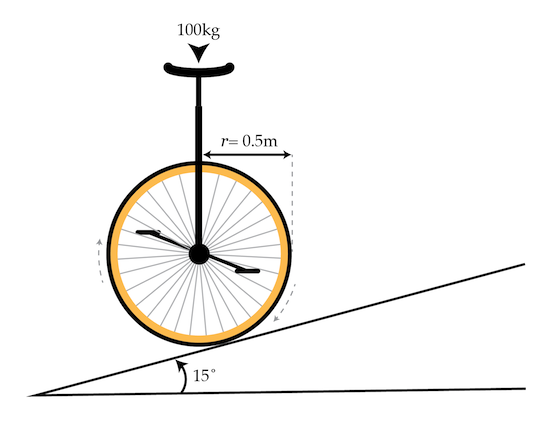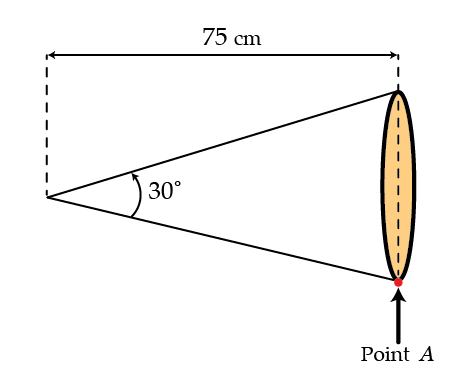Classical Mechanics

# Torque - EquilibriumThree objects are hanging on a scale system, as shown in the figure above. The distances between the objects and the pivots satisfy $r_1=4\text{ m}, r_2=8\text{ m}, r_3=20\text{ m}, r_4=8\text{ m}.$ If the weight of object $A$ is $w_A = 12 \text{ N}$ and the whole scale system is in balance, how much does object $C$ weigh?Five forces $\begin{array}{c}&F_1, &F_2 = 6 \text{ N}, &F_3 = 3 \text{ N}, &F_4 = 6 \text{ N}, &F_5 \end{array}$ are exerted perpendicularly on a rod of length $l,$ as shown in figure above. If the rod is in static equilibrium, what is the magnitude of $F_5?$

A fastener is a system of 2 objects - a bolt and a nut. You come across such a bolt/nut system tightened all the way, so that the nut and the top of the bolt are pressing against each other with a force of 5 N. If the nut is held fixed, how much torque in mJ does one need to put on the bolt to begin to unscrew it?

Details and assumptions

• The radius of the bolt is $R = 2$ mm.
• The threads on the bolt are such that the bolt needs to rotate N = 20 cycles to move $d = 1~cm$ vertically away from the nut.
• The friction coefficient between the bolt and nut is $k = 0.5$.

Between doing physics problems on Brilliant, some people like to unicycle. A unicyclist is cycling up a hill angled $15^\circ$ with respect to the horizontal. The center of mass of the cyclist is directly over the axle of the wheel and the cyclist/unicycle system have a combined mass of $\SI{100}{\kilo\gram}.$ The radius of the wheel is $\SI{0.5}{\meter}$ and the coefficient of static friction between the wheel and the asphalt is $1.$

What is the magnitude of the torque (in $\si{\newton\meter}$) that the cyclist needs to exert on the pedals in order to cycle up the hill at a constant speed?Details and assumptions

• The unicycle does not slip against the hill.
• You may take the acceleration of gravity to be $-\SI[per-mode=symbol]{9.8}{\meter\per\second\squared}.$
• You may neglect air resistance.

Cheerleaders at sporting events will often use a bullhorn to make themselves louder. A bullhorn is simply a hollowed out cone - you speak into the narrow end and your voice comes out the wide end, channeled by the cone so it is louder than it would be otherwise. At a sporting event, a cheerleader holds a bullhorn up to their mouth as in the figure below. The very tip of the bullhorn rests on their mouth and they use their hand on the other end to hold the bullhorn horizontal and still. We model the bullhorn as the rigid 2-d hollow cone of horizontal length $0.75~\mbox{m}$, opening angle $30^\circ$, and (surface) mass density of $0.4~\mbox{kg/m}^2$ (see figure). How much force in Newtons does the cheerleader exert at point A?Details and assumptions

• You may take $g$ to be $9.8~\mbox{m/s}^2$.
• Assume the force at point A is directed straight up.
×